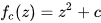## Fractal Universe

Norman Page wrote:

https://rloldershaw.people.amherst.edu/newdevyear/2008/March.htm#:~:text=THE%20MEANING%20OF%20PLANCK’S%20CONSTANT&text=(6)%20makes%20it%20explicit%20that,action%20for%20Atomic%20Scale%20systems.

The universe is fractal at the Plank constants. The best example is the Mandelbrot set which becomes degenerate after iterations down to the Plank constantsI think this means that in the Bessel functions the Y axis leaves the photon with mass and 3 D extension. and the x axis goes to a finite limit in time.
This is indeed an interesting article. Defining scales of similarity seems to work, at least in certain cases. I do not agree on some points, for example: on quantum level of atoms and molecules, there is no predominance of gravitation as is claimed by the inverse fine structure constant of 1/alpha=137. The Schrödinger and Dirac equation are purely electromagnetic and work nearly perfectly. On sub-atomic level the claim may be true. We have used m theory in some papers on this level and obtained encouraging results. Oldershaw takes mini-black holes for qualitative argument, lacking better theories.
Interpreting wave function densities as “subquantum scale plasma particles” may be not so wrong. This is what we call aether or vacuum structure, and I am convinced that on the Planck scale, aether is built from discrete mini-particles. Dynamic processes (for exampel how a pointlike electron becomes an extended wave structure) may be explainable by ECE dynamics. ECE theory provides many new means to investigate suche effects closer.

## Appreciation of new ECE results on the structure of light

Gareth John Evans wrote:
This is a remarkable paper Horst. The appearance of the Golden Ratio in this way (with rest energy increasing by the Golden Ratio) and the establishment that light can escape from a star (with the fundamental confirmation it provides that there are no such things as black holes in the accepted sense) are discoveries of massive significance.
You have traced this back to its core at the fundamental physical level. This is ground breaking in every sense. What a legacy Myron has left and how fitting it is that you, who played such a key role in developing the new physics with him, have observed and now developed this remarkable next step.
“Many congratulations” as Myron would have said!!
ECE theory is even more firmly cemented now and how close Myron was to observing it.

## Deflection of light: a completely new method of calculation

In UFT 442 we handle the deflection of light grazing the sun. With special relativity, the dynamics of such a trajectory cannot be computed, because the gamma factor diverges for v –> c. Within m theory, however, v=c is possible for m>1. An analytical calculation for light corpuscula moving at this speed gives the astonishing result that m is the “golden ratio” number of about 1.6. This is quite high. For ordinary matter (S2 star for example) we found m approx. 1. This leads us to the statement that light (or e-m radiation in general) has a different interaction with the spacetime or background field than dense matter. The mass of the photon seems to be extended in any form so that space appears “less dense” than for ordinary matter.

Since m theory allows velocities of v=c, we are able to apply the Lagrange (or identical Evans-Eckardt) equations to motion of light corpuscula. We can compute the dynamic behaviour of light in the presence of heavy masses. This is possible only by m theory. I did this for several values of a constant m. The result is that the experimental deflection angle is obtained within 2% with the value of the golden ratio. This is a very remakable result, proving the consistency of the calculations. This is a new milestone in theoretical physics.

The fact, that the m value for light equals the golden ratio, is probably the first time where this important constant of natural philosophy was derived from a principal physical theory without assuming any “philosophical principles”.

## New results of m theory for dark stars and escape velocities

Myron’s last paper, for which he wrote notes, was paper 438 (old numeration), which was about dark stars and escape velocities. I am preparing this paper as “opus postumum” of Myron, which will go as UFT 442 to the web site. There are 4 notes of Myron, where he described escape velocities for Newtonian physics, special relativity and m theory. The latter is the highest current standard of general relativity. I extended the calculation – according to Myron’s last statement – to dynamical solutions of the Lagrange equations of m theory. From earlier calculations we thought that these equations are a bit different from the direct solution of energy and momentum conservation, which we called Evans-Eckardt equations. Now I found out (by a different Maxima code solving the same Evans-Eckardt equations) that both equation sets are identical. It is not clear where this difference came from but the result is much more satisfactory than before and earlier problems were resolved.

The escape velocity of an orbiting mass from a gravitating mass is
v = m(r) c,
where r is the starting radius of the escaping mass. Obviously, the velocity of light c is not a fixed escape limit. If m(r)<1, then an object can escape from a heavy star with sub-luminal velocity. In the next post and in paper 442 we will see that this is could be the case for light.